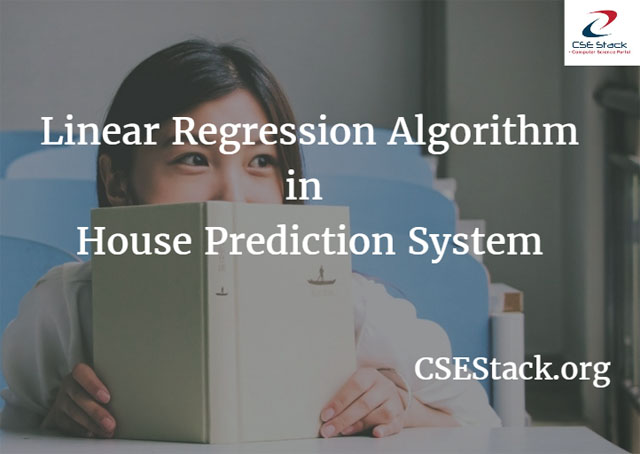# Linear Regression Real Life Example (House Prediction System) Equation

In this tutorial, you will learn about linear regression algorithm. I will also explain Linear Regression real life example. This will make it easy to understand.

### What is a Linear Regression in Machine Learning (ML)?

Linear regression is one of the most popular algorithms in ML.

Linear regression algorithm is used to predict the continuous-valued output from a labeled training set i.e. it is a supervised learning algorithm.

It is a well-known algorithm for machine learning as well as it is well-known in Statistics.

#### Linear Regression Real Life Example

For further explanation, let us consider a Linear Regression example.

We are having a training set of a House prediction system. We are using features of House i.e. area, the number of bedrooms, etc. as input and its price is our output.### How does Linear Regression Algorithm Functions?

1. We are having labeled training set of houses where the input is a matrix having ‘m’ rows and ‘n’ columns. ‘m’ correspond to the number of houses in the training set, each having ‘n’ number of features. The output of this training set is a vector having size ‘m’.

We will use ‘i’ as index denoting row number and ‘j’ as index denoting column number. ‘i’ denotes ith training example and ‘j’ denotes jth feature.

2. Linear regression algorithm is fed with this training set which outputs the function ‘h’. ‘h’ stands for a hypothesis.

So now when ‘h’ is fed with new input it tries to predict its output. Thus ‘h’ is a function that maps X to Y.

#### Linear Regression Equation / Formula

```Y = h(X)
h(X) = a1x1 + a2x2+ … + aNxN
x1, x2, x3, … xN are featured.
For e.g. x1 = size of house,
x2 = number of bedrooms,
x3 = number of bathrooms, etc.
a1, a2, a3, ...aN are coefficients.
Normally ‘theta’ is used as coefficients,
just for my convenience I have used ‘a’ instead of ‘theta’.
Y is price of house.```

3. Now we need to find values of coefficients so that we can use those values to predict output for corresponding input. That is, we need to find values of a1, a2, a3, … aN. For finding values of coefficients we use GRADIENT DESCENT ALGORITHM.

4. Once we have values of all coefficients we can predict the output of linear regression real life example for unseen inputs.

#### How does Gradient descent algorithm function?

1. Gradient descent is an optimization algorithm, where we try to optimize the value of coefficients by minimizing a cost function (cost).

2. Cost function (cost) is having coefficients as parameters. It has a shape like a bowl, where any point on the bowl can be imagined as having coefficients as its coordinates. So minimum value of cost function will be at the bottom of the bowl so there we will have best sets of coefficients.

3. We initialize coefficients with random values. Cost is calculated by plugging that values of coefficients into a function ‘f’.

`Cost = f(coefficient)`

4. Then we calculate a derivative of a cost function, since derivative gives slope at a point, pointing to the direction in which we can move coefficient value in order to get the lower cost in next iteration.

`Delta = derivative(cost)`

5. Once we know which direction is downhill we can keep updating the value of coefficients until the derivative of cost function tends to zero. A learning rate parameter (alpha) is used to control change in the coefficient on each update.

`Coefficient = coefficient – (alpha * delta)`

6. In order to speed up the process of calculation of coefficients do scaling of features i.e. try to get the value of all features in a single range. For e.g. -1 < feature < 1.

So if our feature is the size of the house, we will do scaling of it by dividing each value by 5000(range of size of the house). Therefore if the original size of the house is 2000, we will assume 2000/5000 as our value of the new feature.

This is simple linear regression real life example to get a clear understanding. It is very useful for machine learning.